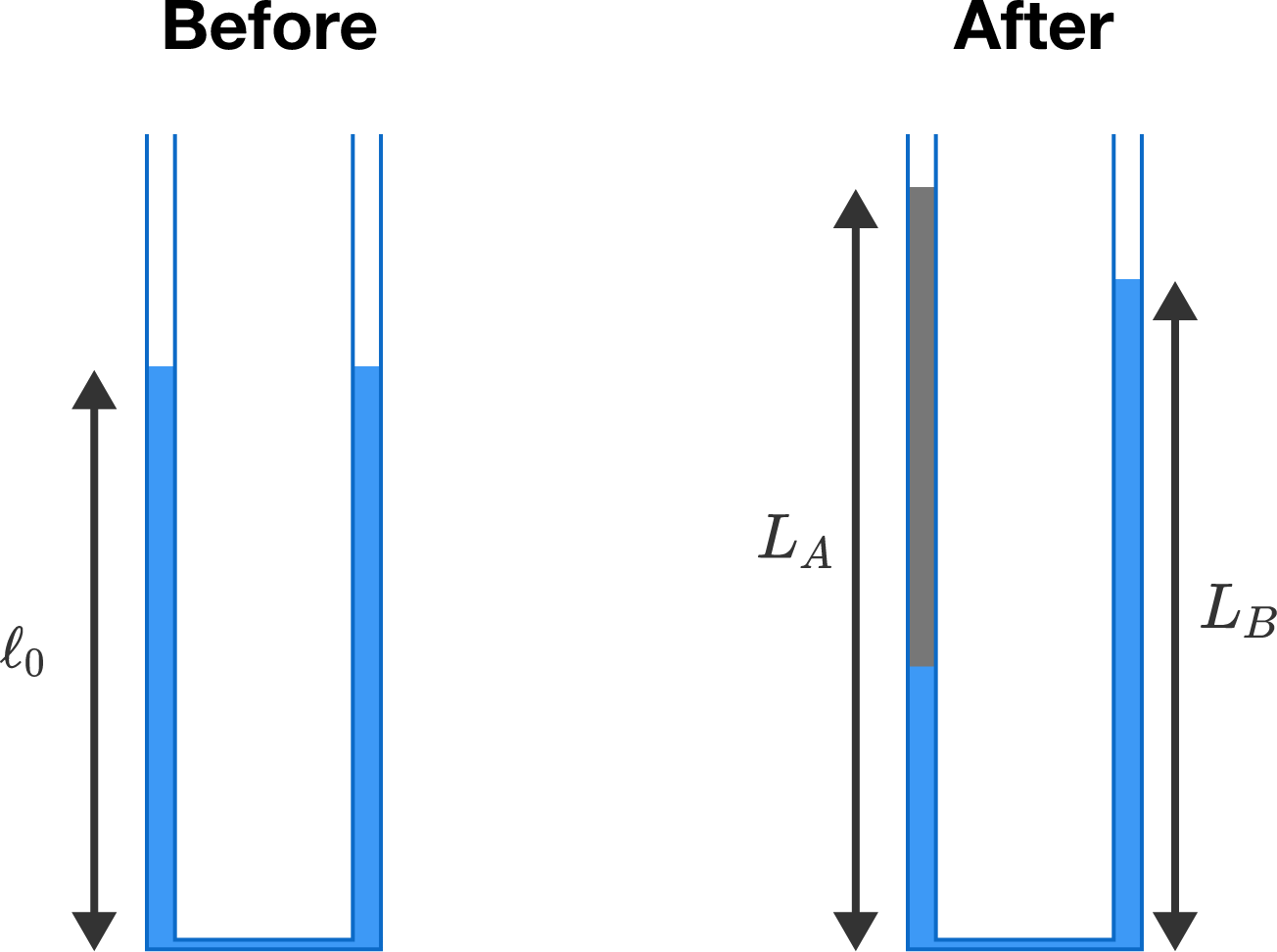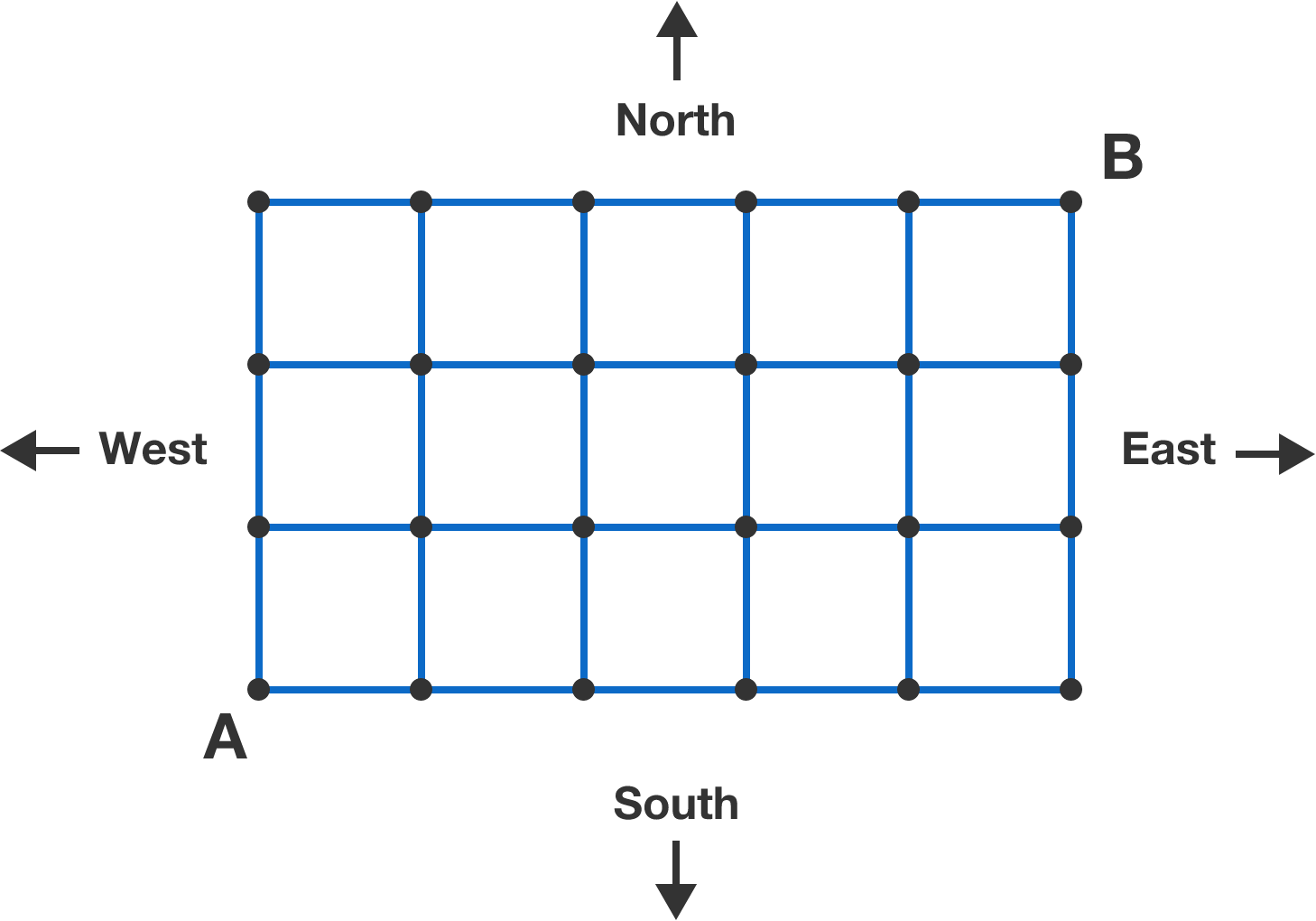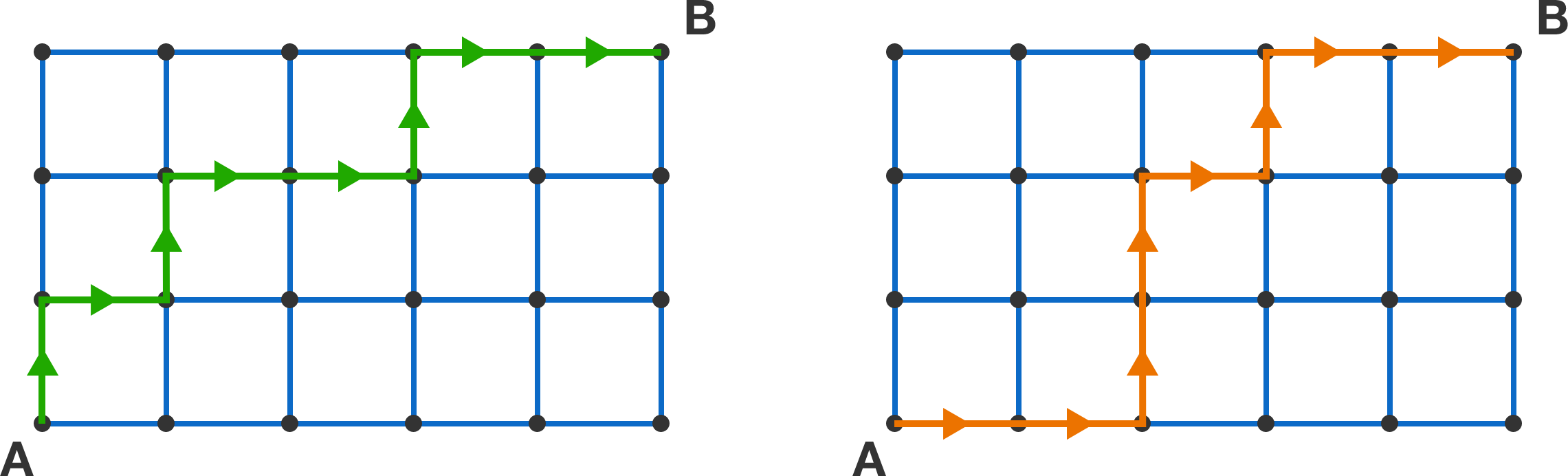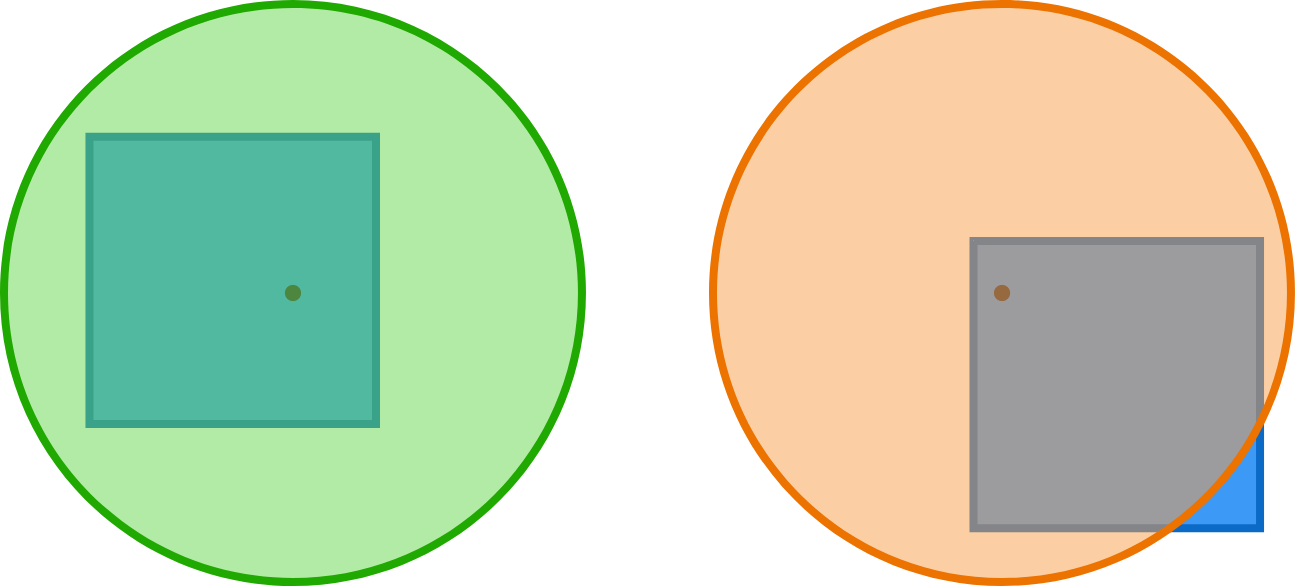# Problems of the Week

Contribute a problem

# 2017-11-20 IntermediateInitially, the U-tube shown above is filled with water, and the level on each side is $$\ell_0 = \SI{5}{\centi\meter}.$$ After some oil is poured on side $$A,$$ the level on that side becomes $$L_A = \SI{7.3}{\centi\meter}.$$

What is $$L_B$$ $$($$in $$\si{\centi\meter})?$$

Assume that the water and oil do not mix, and that their densities are related by $$\rho_\textrm{oil} = 0.85\rho_\textrm{water}.$$

True or False?

For all reals $$k,$$ there exist reals $$x,y,$$ and $$z$$ satisfying $\frac{x}{y+z}+\frac{y}{z+x}+\frac{z}{x+y}=k.$

Find the smallest positive integer which ends in 17, is divisible by 17, and whose digits sum to 17.

I will walk from A to B moving only North and East along the grid lines. Then, I will walk back to A along the gridlines, visiting only locations that I have not visited before.

How many paths are there from A to B that allow me to walk back to A in this way?The green path on the left allows me to walk back to A visiting only new locations, but the orange path on the right does not.

Select a random point from the interior of a unit square (uniformly across the area). Let $$p$$ be the probability that a unit circle centered at that point will completely cover the square.

To three decimal places, what is $$p?$$On the left, the unit circle completely covers the unit square. On the right, the unit circle does not completely cover the unit square.

×

Problem Loading...

Note Loading...

Set Loading...